Author: Oscar Cronquist Article last updated on February 03, 2019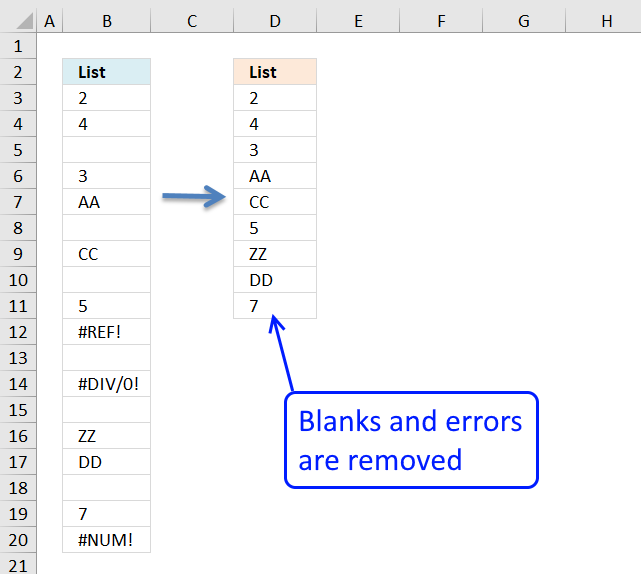In this blog post I will provide two solutions on how to remove blank cells and a solution on how to remove blank rows:

### Remove blank cells [array formula]

Column B is the list with random blank cells. Column D is the list without the blank cells.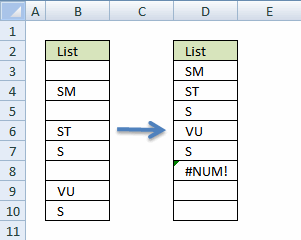Array formula in cell D3:

=INDEX(\$B\$3:\$B\$10, SMALL(IF(ISBLANK(\$B\$3:\$B\$10), "", ROW(\$B\$3:\$B\$10)-MIN(ROW(\$B\$3:\$B\$10))+1), ROW(A1)))

Recommended article

Delete blanks and errors in a list

The formula deletes blank cells and cells with errors. It doesn't matter if the cells contain numbers or text, they […]

#### How to create an array formula

1. Copy (Ctrl + c) and paste (Ctrl + v) array formula into formula bar. See picture below.
2. Press and hold Ctrl + Shift.
1. Press Enter once.
3. Release all keys.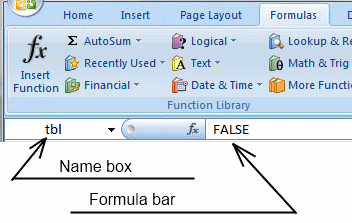How to enter an array formula

Array formulas allows you to do advanced calculations not possible with regular formulas.

#### How to copy array formula

1. Copy (Ctrl + c) cell D3
2. Paste (Ctrl + v) array formula on cell range D3:D8

#### Remove #num errors (excel 2007)

When you run out of values to show, the array formula above returns #NUM! errors. You can avoid this if you use the IFERROR function, however use it with great caution. It not only finds #NUM! errors but all errors. So if you formula or cells contain an error you won't see it, the IFERROR function removes that error too.

=IFERROR(INDEX(\$B\$3:\$B\$10, SMALL(IF(ISBLANK(\$B\$3:\$B\$10), "", ROW(\$B\$3:\$B\$10)-MIN(ROW(\$B\$3:\$B\$10))+1), ROW(A1))), "")

How to use the IFERROR function

If the value argument returns an error, the value_if_error argument is used. If the value argument does NOT return an error, the IFERROR function […]

* You will also get a weekly newsletter, unsubscribe anytime!

Recommended article

### Remove blank cells [keyboard shortcut F5]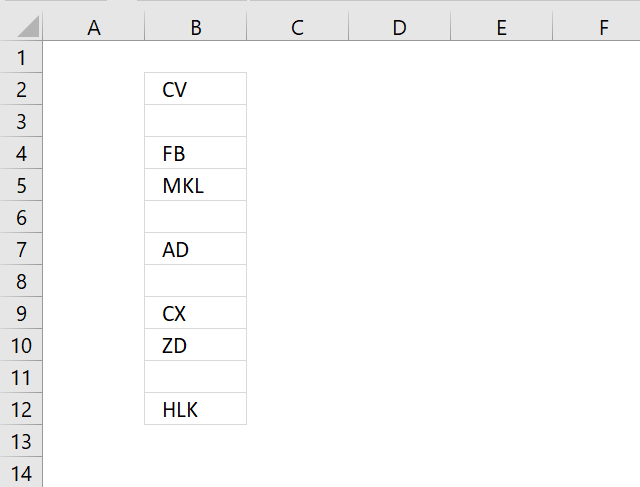The image above shows random cell values in column B, follow these simple steps to remove blank cells in column B.

1. Select range B2:B12.
2. Press F5 and a dialog box appears.3. Click "Special..." button.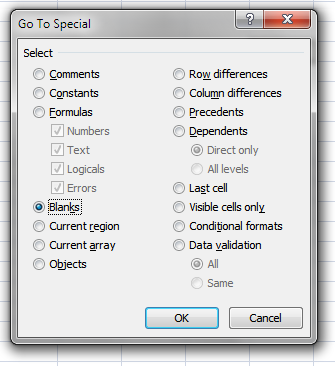5. Click OK button. The image below shows that the cell range selection changed, now only blank cells are selected.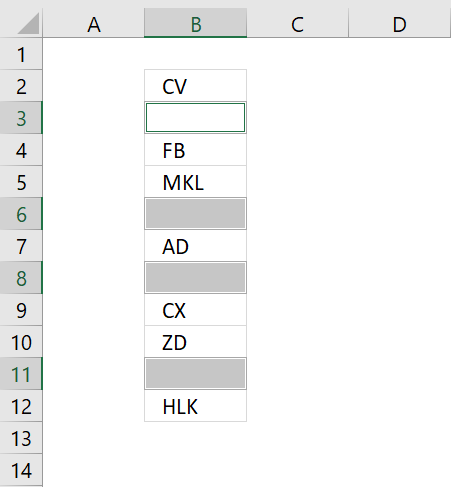6. Right-click on one of the selected blank cells and a context menu appears, select "Delete..".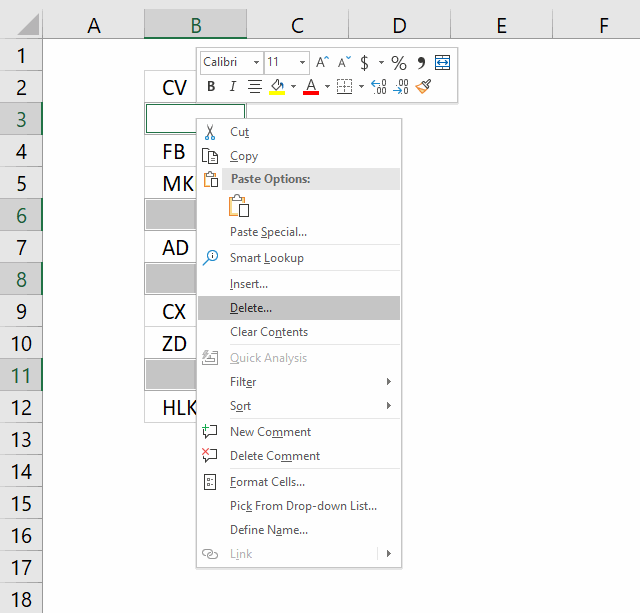7. Another dialog box appears, click "Shift cells up".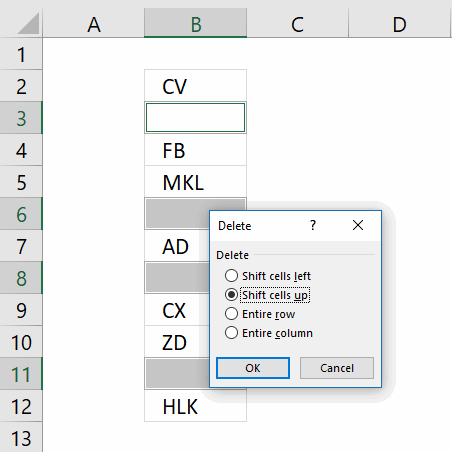"Shift cells up" will delete selected blank cells and move non empty cells up. This step will mess up your dataset if you have values arranged as records.
"Entire row" will delete row 3, 6, 8 and 11 in image above. If you have data on these rows they will be deleted as well.
8. Click OK button.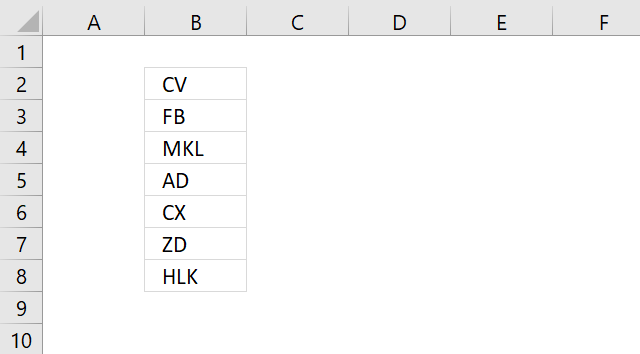The image above shows that blank cells are now deleted.

### Remove blank rows (array formula)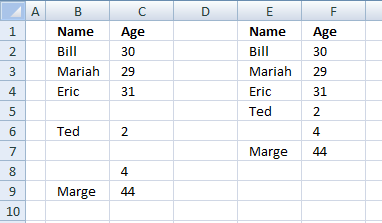Array formula in cell E2:

=IFERROR(INDEX(List, SMALL(IF(FREQUENCY(IF(List<>"", MATCH(ROW(List), ROW(List)), ""), MATCH(ROW(List), ROW(List)))>0, MATCH(ROW(List), ROW(List)), ""), ROW(A1)), COLUMN(A1)), "")

#### How to create an array formula

1. Select cell E2
2. Paste formula
3. Press and hold Ctrl + Shift
4. Press Enter

#### How to copy array formula

1. Select cell E2
2. Copy cell (Ctrl +c)
3. Select cell range E2:E10
4. Paste